Hill Analysis of Interactive Ligand Binding (lig)

In this exercise, the ligand binding interactions between the bivalent ligand binding sites of symmetrical dicarboxylic acid are examined using a Hill plot. When fully protonated ("saturated"), a dicarboxylic acid undergoes a two-step dissociation reaction with decreasing hydronium ion concentration. When completely deprotonated ("desaturated"), a dicarboxylate undergoes a two-step association reaction with increasing hydronium ion concentration.

For correct statistical analysis is it necessary to keep track of binding to the right and left sides of the molecule.

Two-step dissociation
kd1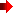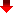HOOC-CH2-COOH
 - H+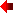+H+
-OOC-CH2-COOHkd1'ka2kd2
 - H+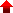+ H+ka2'ka1+ H+ - H+ka1'HOOC-CH2-COO-+H+ - H+-OOC-CH2-COO-kd2'
Two-step association
 kd1-cooh = [H+] * [-OOC-CH2-COOH] / [HOOC-CH2-COOH] (step d1) kd1'-cooh = [H+] * [HOOC-CH2-COO-] / [HOOC-CH2-COOH] (step d1') kd2-cooh = [H+] * [-OOC-CH2-COO-] / [-OOC-CH2-COOH] (step d2) kd2'-cooh = [H+] * [-OOC-CH2-COO-] / [HOOC-CH2-COO-] (step d2') kd1 = kd1' = 1/ka2 = 1/ka2' kd2 = kd2' =1/ka1 = 1/ka1' ka1-cooh = [-OOC-CH2-COOH] / [ H+] * [-OOC-CH2-COO-] (step a1) ka1'-cooh =[HOOC-CH2-COO-] / [H+] * [-OOC-CH2-COO-] (step a1') ka2-cooh = [HOOC-CH2-COOH] / [H+] * [-OOC-CH2-COOH] (step a2) ka2'-cooh = [HOOC-CH2-COOH] / [H+] * [HOOC-CH2-COO-] (step a2') ka1 = ka1' = 1/kd2 = 1/kd2' ka2 = ka2' = 1/kd1 = 1/kd1'

Continue the acid-base titration analysis of dicarboxylic acid by opening the Excel spreadsheet below.

• Up and down arrow dials adjust the pH1/2, i.e., the midpoint, and the slope of a straight line.

Dicarboxylic Acid Titration: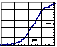Hill PlotReturn to Biochemistry 108A Home PagePress the browser's "Back" key to return to previous page.Search for a Specific Topic